# Full-Wave Rectifier

Rectifiers are circuits that convert alternating current (AC) into direct current (DC). Rectifiers are very common in electronics because most electronic devices use DC, but the power grid (mains electricity) supplies AC. The three most common types are half-wave, full-wave, and bridge rectifiers.

A full-wave rectifier is a circuit that allows a complete alternating current (AC) waveform to pass, turning an AC signal into a pulsed direct current (DC) signal.

In contrast, half-wave rectifiers allow only one half (the positive half) of an AC waveform to pass. The negative part of the AC waveform is essentially wasted. By using the full AC input wave, a full-wave rectifier (FWR) is twice as efficient as a half-wave rectifier and produces a higher quality DC signal.

However a full-wave rectifier uses two diodes where a half-wave rectifier requires only one, and an FWR also requires a larger/more complex transformer known as a center tapped transformer.

The bridge rectifier is another common rectifier that also passes a complete AC waveform and is also occasionally referred to as a type of full-wave rectifier as a result.

Bridge rectifiers are more complex than traditional FWR’s and require four (4) diodes to function, but they can be used with a normal transformer whereas an FWR requires a center tapped transformer and are therefore often cheaper.

The following table provides a comparison of each type of rectifier.

## Full-Wave Rectifier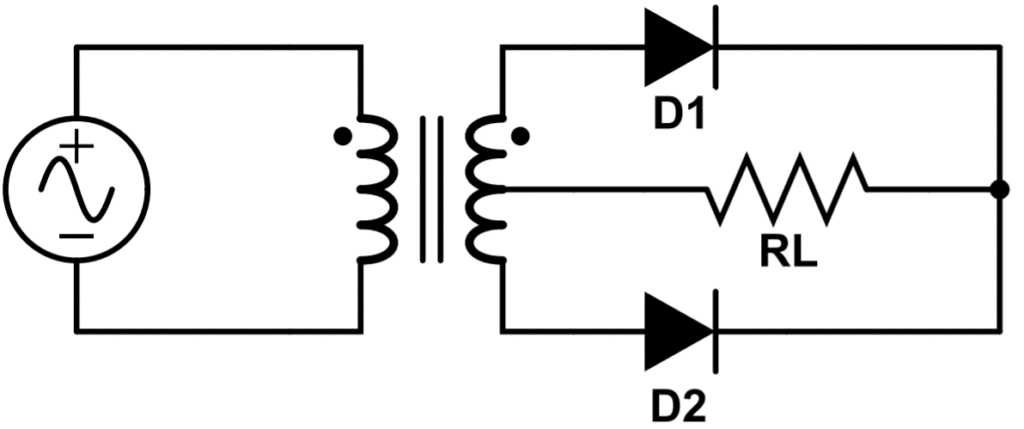A full-wave rectifier converts an AC waveform into DC. It consists of a center tapped transformer and two diodes. The output is measured across the load (RL).

Full-wave rectifiers build on the structure of half-wave rectifiers, and can be thought of as a modified version of two half-wave rectifiers stacked together.

In a full-wave rectifier, one diode (D1) rectifies the positive part of the AC input just like a half-wave rectifier. The output of this diode is identical to the output of a half wave rectifier.

However, a second diode (D2) is also used in order to rectify the negative part of the input AC waveform. The output of D2 is identical to the output of D1 but its’ pulses occur while the output of D1 is zero. This results in double the number of output pulses produced by a half-wave rectifier.

There is one complication in going from a half-wave rectifier to a full-wave rectifier. The negative part of the AC waveform must be inverted to produce this second positive pulse in order to achieve the final waveform output of the FWR.

A center tapped transformer is used to invert the AC waveform before sending it through diode D2.

This is accomplished by connecting the cathode of the diode to the center-tap of the transformer.

The result is an output waveform that features continuous pulses.

This is a significant improvement over the output of an HWR, whose waveform features pulses separated by equal periods where the voltage equals zero (0).

## Full-Wave Rectifier Circuit

Like half-wave and bridge rectifiers, full-wave rectifiers rely on the functionality of diodes. Diodes only allow electric current to flow in only one direction, based on the operation of semiconductor p-n junctions. Current in the diode can only flow from the anode to the cathode:

Current can only flow from the anode to the cathode; it can’t flow in the reverse direction without harming the diode. This is the primary feature that is used to convert AC into DC.

Full-wave rectifiers use two diodes to convert an AC input into a DC output. One diode rectifies the one part of the AC waveform, and the second diode rectifies the second part of the AC waveform.

### FWR and Center Tapped Transformer

Unlike a half-wave rectifier (HWR), a full-wave rectifier (FWR) requires a center tapped transformer in order to function.

This specialized transformer allows us to make use of the negative part of the AC input waveform that would normally go unused in a half-wave rectifier.

Let’s see how this works.

Standard transformers are used in both half-wave and bridge rectifier circuits.

They have one primary winding and one secondary winding. There are two connection points on both windings where wires are attached to connect the transformer to a circuit. The output voltage VO is measured across the entire secondary winding. This type of transformer is used in half-wave and bridge rectifiers.

#### Standard Transformer

In contrast, center tapped transformers have three connection points on the secondary windings. There is one on each end of the transformer, and one right in the middle of the windings, called a center tap.

#### Center Tapped Transformer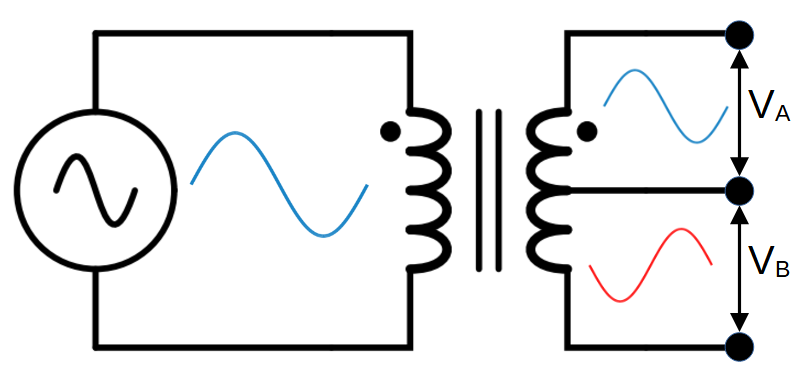The input waveform is transformed into two output waveforms. VA (in blue) is in phase with the input waveform, but VB (in red) is inverted.

This connection effectively splits the transformer windings in half, creating two sides of the transformer that can each be used as independent voltage sources, VA and VB.

The center tap allows the transformer to function as an inverter by producing an output AC waveform that is inverted, or phase shifted by 180°.

The ‘inverting action’ occurs automatically when the center tapped transformer is connected properly, with the center tap functioning as the ground connection for both VA and VB.

Like the standard transformer used in an HWR, the center tapped transformer in a FWR is also used to step-down the voltage from a standard AC input.

There is one caveat to the usage of a center tapped transformer, however.

Because only half of the windings can be used to produce the output voltage, a center tapped transformer must have twice the number of windings as an equivalent standard transformer in order to achieve the same voltage output. This makes the FWR more expensive than a HWR.

### Full-Wave Rectifier Circuit DiagramA full-wave rectifier consisting of a center tapped transformer and two diodes. The output voltage is measured across the resistive load RL.

Despite the added complexity of the center tapped transformer, full wave rectifiers feature a relatively simple design that is very similar to that of the half wave rectifier.

Each of the two diodes will only allow current to pass when they are forward biased.

The total output of the circuit, Vout, is measured across the load RL.

Since a diode only allows current to flow in one direction, when it is connected with an alternating current (AC), it will only allow the ‘positive’ current to pass. This produces a type of DC current known as pulsed DC.

Due to the waveform inversion from the center tapped transformer, each diode rectifies the input waveform alternately, with each producing a positive pulse in turn.

The following image shows an identical circuit that has been rearranged to show that the center tap of the transformer is connected to the cathode (negative terminal) of RL, and are typically grounded (the triangle symbol represents ground).

## Full-Wave Rectifier Operation

### How Does a Full-Wave Rectifier Work?

Direct current (DC) always flows in one direction, but alternating current (AC) flows in both directions in a sinusoidal pattern, called a waveform.

The image on the right shows the waveform of 120V AC power in the US, which has a frequency of 60 Hz.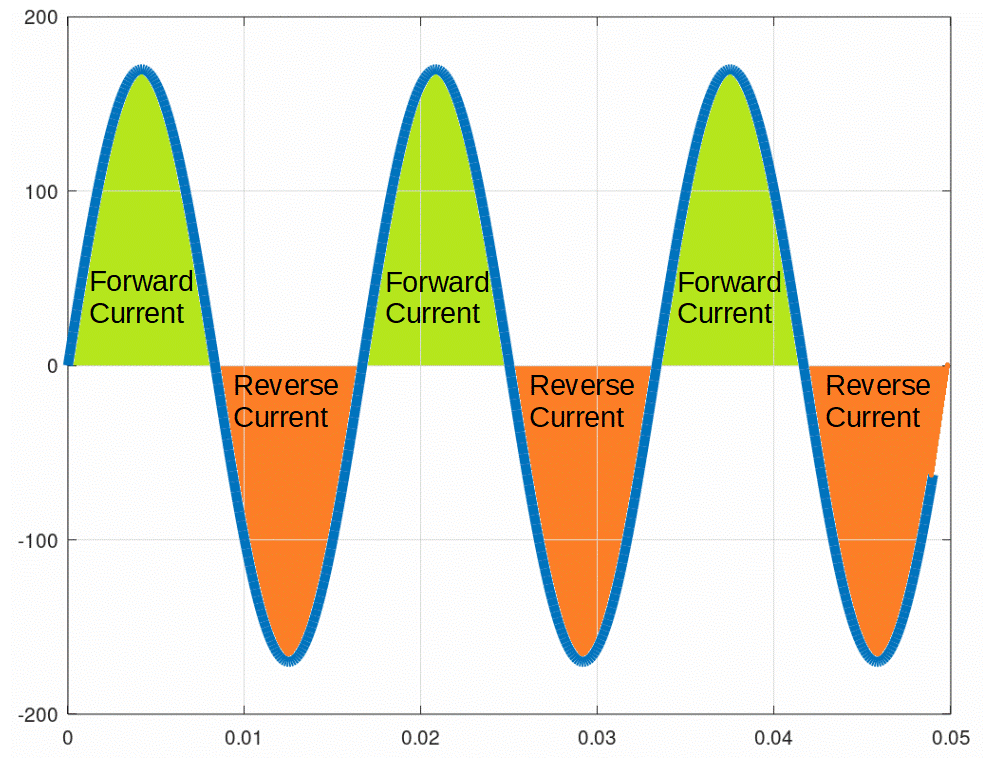Sine wave showing the directionality of current with positive and negative voltage. When the voltage is positive, the current moves in the ‘forward’ direction. When the voltage is negative, the current moves in the ‘reverse’ direction.

When the waveform is positive, the current is moving in the ‘forward’ direction.

When the waveform is negative, the current is moving in the ‘reverse’ direction.

This is why this type of current is called alternating current; the current alternates direction. Instead of electrons processing through a circuit, they wiggle back and forth in the opposite direction of conventional current.

Each diode in a full-wave rectifier is used to allow only the positive current to flow.

The diodes remove the negative part of the AC waveform, ‘chopping off’ the bottom half wave of the AC signal and leaving only the top half wave.

This results in a pulsed DC signal that retains only the positive part of the AC waveform.

In the pulsed DC output of the half-wave rectifier, current always moves in the same direction, but increases and decreases over time, with periods of zero (0) current in between pulses.

In a full-wave rectifier, however, the goal is to make use of the entire input AC waveform rather than just wasting the negative half as a half-wave rectifier does.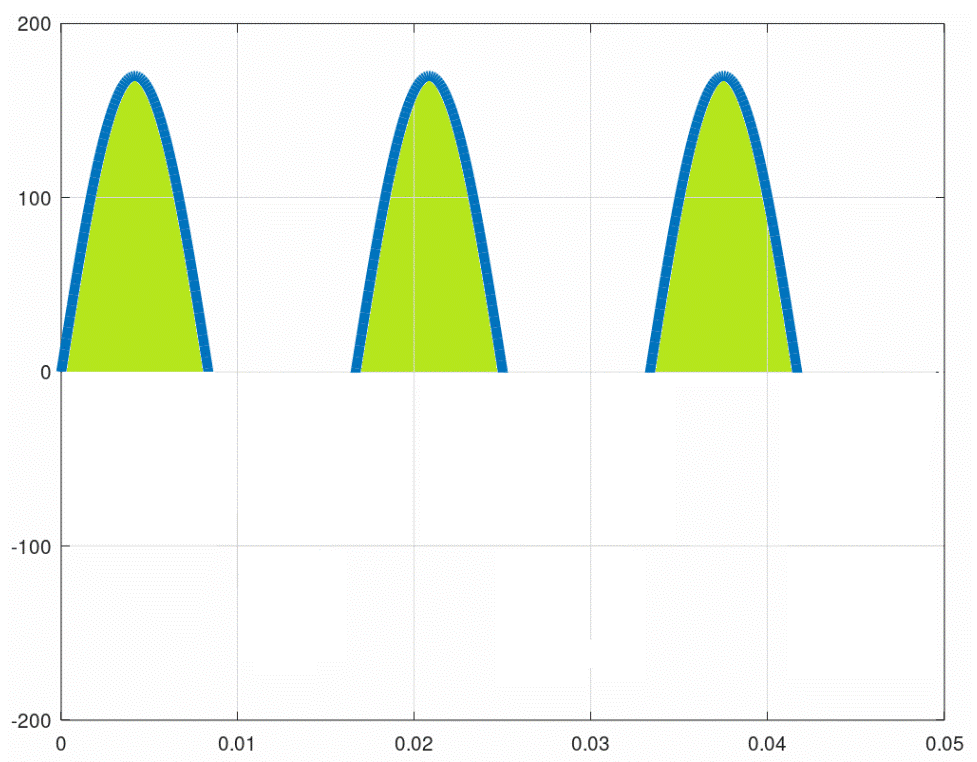The pulsed DC output of a half-wave rectifier. The rectifier filters out the negative part of the AC waveform, leaving only positive pulses.

This requires a slightly more complex process.

There are three steps to the rectification process of a full-wave rectifier:

1) The center tapped transformer produces two voltages, VA and VB. VA is in phase with the input AC waveform, and VB is 180 degrees out of phase with the input AC waveform.

#### Output of Diode D1

2) Diode D1 rectifies VA from the transformer, producing a pulsed DC output identical to the output of a half-wave rectifier.

This output features periods with a positive pulse and equal periods during which the output is zero (0).

3) Diode D2 rectifies VB from the transformer, producing a second pulsed DC output that is identical to the output of D1 but is phase shifted by 180 degrees.

This means that as D1 is pulsing, D2 is ‘off’. As D2 is pulsing, D1 is ‘off’.

#### Output of Diode D2

The output of D2 is then combined with the output of diode D1, forming a waveform with constant pulses:

Note that the blue and red colors are only there to help you visualize this process. The actual waveform is simply the shape, i.e. there is no significance to the alternating blue and red peaks other than showing that the blue peaks were contributed by diode D1 and the red peaks were contributed by diode D2.

Comparing the output of a full-wave rectifier with that of a half-wave rectifier, we can see that the gaps between pulses have been eliminated. This is the main advantage of the full-wave rectifier. Thus the full-wave rectifier is more efficient and also produces a higher quality DC output than the half-wave rectifier.

## Full-Wave Rectifier Formula

The equivalent DC voltage output of a full-wave rectifier is the average value of the voltage pulse.

This can be found by using the peak voltage (Vpeak) in the following formula:

V_{DC} = \frac{2V_{peak}}{\pi}

Note: Deriving this formula requires some calculus. You can find the derivation at the end of this tutorial if you’re interested.

However, the peak voltage isn’t exactly the peak of the AC voltage input. The diode has a voltage drop called the forward voltage. For silicon-based diodes, the voltage drop is about .7 volts. So Vpeak is equal to the peak AC voltage minus the forward voltage of the diode:

V_{peak} = V_{ACpeak} - .7 V

Therefore the average DC output voltage can be related directly to the peak of the AC waveform:

V_{DC} = \frac{2(V_{ACpeak}-.7V)}{\pi}

## Full-Wave Rectifier With Capacitor Filter

The full-wave rectifier produces a higher quality output signal than the half-wave rectifier. However it still features pulses that decline all the way to zero and then rise back up the peak.

Like half-wave rectifiers, the output of the full-wave rectifier can be significantly improved by adding a capacitor to the circuit.

### Full-Wave Rectifier Capacitor Filter Circuit Diagram

The capacitor stores charge when the voltage is increasing during the ‘upward’ section of the wave. A corresponding voltage is generated across the capacitor.

When the voltage begins to decrease, the capacitor begins to act as a second voltage source, releasing the charge it has stored.

Instead of dropping to zero, the new waveform slowly declines from the peak voltage as the capacitor discharges.

Thus the capacitor buffers the total voltage measured across the load.

The capacitor then recharges during the next cycle, and the process begins again.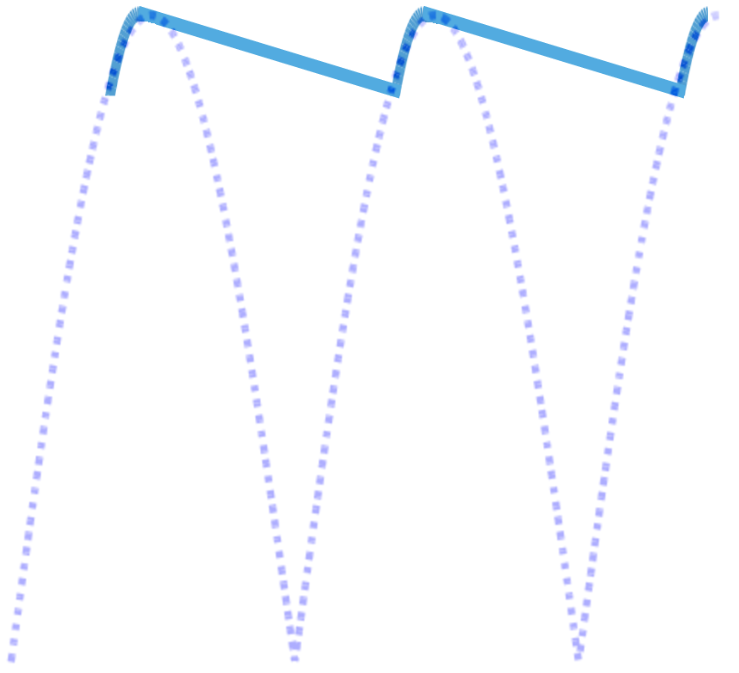The output of a full-wave rectifier with a capacitor filter. The dashed curve represents the output of the rectifier without a capacitor. The solid line represents the improved waveform due to the inclusion of a capacitor.

This results in a waveform that much more closely resembles an ideal DC signal, which would be a flat line.

## Advantages and Drawbacks of Full-Wave Rectifiers

Full-wave rectifiers are the simplest way to achieve rectification of a full AC waveform.

Compared with half-wave rectifiers, the output signal is of a much higher quality. When a capacitor is added to the circuit, the final waveform approaches something resembling an ideal, ‘flat’ DC waveform.

In addition, the efficiency is double that of a half-wave rectifier because both the positive and negative parts of the AC input waveform are used.

However, center tapped transformers are considerably more expensive than regular transformers. Not only are they more complex, the number of secondary windings must be doubled in order to achieve the same voltage at the center tap as a regular transformer achieves across the entire secondary winding.

Since the transformer is likely the most expensive component, this requirement can dramatically increase the overall cost of circuit construction. This may not be significant for prototypes, but it can have a significant influence on any production equipment relying on the circuit.

It turns out that by adding two (2) more diodes and slightly adjusting the configuration, we can make a different type of rectifier called a bridge rectifier. Also called a diode bridge or Graetz circuit, bridge rectifiers eliminate the need for a center-tapped transformer. Since diodes are incredibly cheap, constructing a bridge rectifier is only slightly more expensive than a half-wave rectifier.

## Mathematical Analysis of Full-Wave Rectifiers

The following topics will cover slightly more advanced topics of full-wave rectifiers like current, ripple factor and transformer utilization factor (TUF). While these topics are not crucial for a basic understanding of full-wave rectifiers, we are including them here to function as a comprehensive tutorial and reference.

## Full-Wave Rectifier Formula Derivation

The calculation of the formula for the average output of the full-wave rectifier is identical to that of the half-wave rectifier. The only difference is that the final value is not divided by two (2) because we don’t need to reduce the number of pulses to that of a half wave. So if you already read the tutorial on half-wave rectifiers, what follows will be a review.

The average value of any curve can be found by finding the area under the curve and dividing by the x-axis dimension over which we are trying to calculate the average.

In this case, we are trying to find the average value of the top half of a sine curve, which corresponds to the pulsed DC output of the half-wave rectifier.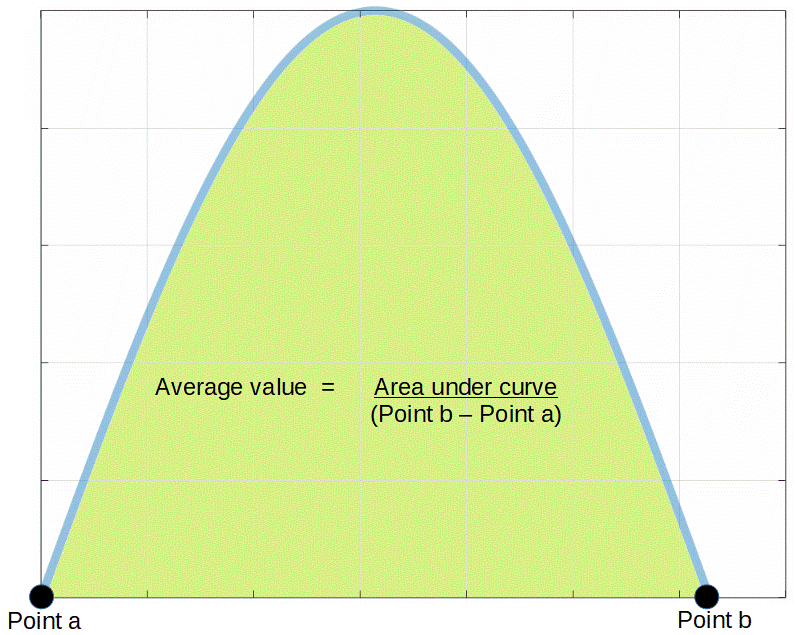An image of a sinusoidal pulse. The average value of the pulse consists of the area divided by the pulse width.

Finding the area under a sine curve isn’t easy using traditional geometrical methods (dividing the curve up into tine rectangles).

Calculus provides a much easier way to find the area under the curve by calculating its’ integral. For example, in order to find the area of the sine wave between point a and point b in the figure, we can simply calculate the definite integral of sine (which is negative cosine) between points a and b:

\int_{a}^{b} \sin x \, dx = - \cos x \rvert_a^b

We scale this result to the value of the peak of the waveform by multiplying it by Vm:

Area =  V_m \int_{a}^{b} \sin{x} \, dx = -V_m \cos{x}\rvert_{a}^{b}

Point a and b are both located where the y-value of the curve (the voltage) is equal to zero. This corresponds with values of zero (0) and pi (π). So we need to evaluate the function between 0 and π:

Area = V_m \int_{0}^{\pi} \sin{x} \, dx = -V_m \cos{x}\rvert_{0}^{\pi}

Now we just need to evaluate cosine at 0 and π and simplify:

Area = -V_m \cos{x}\rvert_{0}^{\pi} = -V_m [\cos \pi - \cos 0] = -V_m [-1-1]=-V_m[-2]=2V_m

So 2Vm is the area under the curve.

In order to calculate the average value, we simply divide this by the x-axis dimensional ‘length’ between points a and b. Point a is at zero and point b is at π so this is equal to π – 0, or π:

Average= V_{DC} = \frac{2V_m}{\pi - 0} = \frac{2V_m}{\pi}

## Current in Full-Wave Rectifiers

We can derive the current in a full-wave rectifier using the same procedure that we used for the half-wave rectifier.

We’ll start by noting that the current in a full-wave rectifier varies periodically (like a sine wave) with the voltage.

Let’s use the term Vi to designate the voltage coming from the secondary windings of the transformer:

V_i = V_{m}\sin{2\pi ft}

We can then use Ohm’s Law to derive the current, and we should note that the current will be limited by two types of resistance: (1) the load resistance RL, and (2) the forward resistance of the diode Rf. The forward resistance can be found by using the diode’s I-V characteristic.

I = \frac{V_i}{R_f+R_L}=\frac{V_{m}}{R_f+R_L}\sin{2\pi ft}

We can also define a new term, Im, again using Ohm’s Law. This will help us simplify this equation a bit and aid in future calculations:

I_m = \frac{V_m}{R_f+R_L}

Therefore in terms of Im, the current is:

I = I_m \sin{2\pi ft}

We can also define another helpful term, α, to simplify this equation even further:

\alpha =2\pi ft

Therefore the current is:

I = I_m \sin{\alpha}

Note that all we’ve done is define the current as a sine wave, and use Im and α to simplify it.

## RMS Current in Full-Wave Rectifier

Another important value is the root mean square (RMS) of the current. The RMS is the square root of the mean, squared (to the second power):

I_{rms} = \sqrt{\frac{1}{2\pi}\int_0^{2\pi}I^2 \,d\alpha}

Not that the factor of 1/2π in front of the integral is used because we are taking the average value over the range of 0 to 2π.

Therefore the value of Irms2 is equal to:

I_{rms}^2 = \frac{1}{2\pi}\int_0^{2\pi}I^2 \,d\alpha = \frac{1}{2\pi}\int_0^{\pi} I_m^2 \sin^2{\alpha} \,d\alpha

Where the term from π to 2π goes to zero because the current is zero for the second half-cycle.

Simplifying this, we find:

I_{rms}^2 = \frac{I_m^2}{2}

Therefore the RMS current is:

I_{rms} = \frac{I_m}{\sqrt2}

## Form Factor of Full-Wave Rectifier

The form factor (abbreviated by f) is a quantity used to help compare the RMS and average values of a function.

It is defined as the ratio of the RMS current over the average current:

f=\frac{I_{rms}}{I_{DC}} = \frac{\frac{I_m}{\sqrt{2}}}{\frac{2I_m}{\pi}} =\frac{\pi}{2\sqrt{2}} \approx 1.1107

## Full-Wave Rectifier AC Output

The total output current can be divided into a DC component and an AC component. The DC component is identical to the average value over the whole waveform, IDC, and we can express that AC component as I’.

I = I_{DC} + I'

Where I’ represents the AC component of the output waveform.

It turns out that the RMS of I’ is an important factor in its’ own right.

We can define I’ as the difference between the total current and the DC component of the current:

I' = I-I_{DC}

We can then find the RMS value of I’ by calculating the square root of the square of its’ mean:

I_{rms}' = \sqrt{\frac{1}{2\pi}\int_0^{2\pi}I'^2 \,d\alpha} = \sqrt{\frac{1}{2\pi}\int_0^{2\pi}(I-I_{DC})^2 \,d\alpha}

Just as we did earlier, we can simplify this by squaring both sides:

I_{rms}'^{2} = \frac{1}{2\pi}\int_0^{2\pi}(I-I_{DC})^2 \,d\alpha = \frac{1}{2\pi}\int_0^{2\pi}I^2-2I(I_{DC})+I_{DC}^2 \,d\alpha

This can be divided into three individual terms. The first is identical to I2rms the second simplifies to -2I2DC and the third simplifies to I2DC.

In other words,

I_{rms}'^{2} = I_{rms}^2 - 2I^2_{DC} +I_{DC}^2 = I_{rms}^2 - I_{DC}^2

Therefore the RMS of the AC component is:

I_{rms}' = \sqrt{I_{rms}^2 - I_{DC}^2}

## Full-Wave Rectifier Ripple Factor

Now that we have quantified the AC component of the full-wave rectifier, we can compare it’s RMS value with the RMS value of the DC component.

This ratio is called the ripple factor, which helps us to understand the magnitude of the AC component compared with the magnitude of the DC component.

The ripple factor is abbreviated by the Greek letter gamma (γ):

\gamma = \frac{I_{rms}'}{I_{DC}}

Using the values we found earlier, we can write the ripple factor of the full-wave rectifier as:

\gamma=\frac{\sqrt{I_{rms}^2-I_{DC}^2}}{I_{DC}}=\sqrt{(\frac{I_{rms}}{I_{DC}})^2-1}=\sqrt{f^2-1}=\sqrt{(\frac{\pi}{2\sqrt2})^2-1} \approx .483

Note that we substituted in the value for the form factor (f), which we previously calculated, to make the final result easier to obtain.

A high ripple factor indicates that the signal still has a large AC component, indicating that the resulting current is far from an ideal DC signal. When we compare this value with that of the half-wave rectifier (ripple factor of ~1.21), we can see that we have achieved a major improvement of the output signal quality.

## Efficiency of Full-Wave Rectifier

The efficiency of the circuit is the measure of its’ power output to its’ power input. Efficiency is abbreviated by the Greek letter eta (η).

To calculate the efficiency, we must find the output power of both the DC and AC components of the output waveform. In other words,

\eta = \frac{P_{O,DC}}{P_{in}}

Where PO,DC is the output DC power and Pin is the input power.

For the DC component, the output power is given by the I2R formula:

P_{O,DC} = I_{DC}V_{DC}=I_{DC}I_{DC}R_L=I_{DC}^2R_L

For the input, we use the relation P = VI:

P_i = V_i \times I = V_m\sin{\alpha}\times I_m\sin{\alpha}=V_mI_m\sin^2{\alpha}

This is the formula for the instantaneous power at a specific value of α; to find the total power, we must integrate:

P_{in}=\frac{1}{2\pi}\int_0^{2\pi}P_i\,d\alpha

Substituting Pi, we find:

P_{in}=\frac{1}{2\pi}\int_0^{\pi}V_mI_m\sin^2{\alpha}\,d\alpha

Noting again that the π to 2π component is again zero as the current is zero.

Recall the definition of Vm:

V_m=I_m(R_f+R_L)

We now substitute this into the equation for Pin:

P_{in}=\frac{1}{2\pi}I_m^2(R_f+R_L)\int_0^{\pi}\sin^2{\alpha}\,d\alpha

Recall the our formula for Irms from earlier:

I_{rms}^2=\frac{1}{2\pi}\int_0^{\pi} I_m^2 \sin^2{\alpha} \,d\alpha

Which we can use to simplify Pin:

P_{in}=I_{rms}^2(R_f+R_L)

We can now solve for the efficiency of the half-wave rectifier:

\eta=\frac{I_{DC}^2R_L}{I_{rms}^2(R_f+R_L)}

Substituting the known values for IDC and Irms:

\eta=\frac{\frac{4I_m^2}{\pi^2}}{\frac{I_m^2}{2}}\frac{R_L}{R_f+R_L}=\frac{8}{\pi^2}\frac{R_L}{R_f+R_L}=0.81\frac{R_L}{R_f+R_L}

Thus we can see that the maximum possible efficiency of the half-wave rectifier is 81%. This is twice the efficiency of the half-wave rectifier.

## Full-Wave Rectifier Transformer Utilization Factor (TUF)

The transformer utilization factor is the ratio of DC output power to the AC rating of the secondary winding.

Mathematically, this can be written as:

TUF = \frac{P_{o,DC}}{2\times V_{RMS}\times I_{RMS}}

The factor of 2 in the denominator comes because the total rating must be twice the value of VRMS times IRMS. This is because the RMS values are taken across half the transformer.

The DC power output can be found by using the I2R formula:

P_{o,DC}=I_{DC}^2R_L

The RMS value of a full sine wave is the peak value of the wave divide by the square root of two (2), so we can state that VRMS must be equal to:

V_{RMS} = \frac{V_m}{\sqrt{2}} = \frac{I_m(R_f+R_L)}{\sqrt{2}}

We have previously found that the RMS value for the current (IRMS) is:

I_{RMS} = \frac{I_m}{2}

Thus the transformer utilization factor is:

TUF = \frac{I_{DC}^2R_L}{2\times \frac{I_m}{\sqrt{2}}\frac{I_m}{2}(R_f+R_L)}= \frac{4I_m^2}{\pi^2}\frac{R_L}{2\times\frac{I_m}{\sqrt{2}}\frac{I_m}{2}(R_f+R_L)}=\frac{4\sqrt{2}}{\pi^2}\frac{R_L}{R_f+R_L}=0.573\frac{R_L}{R_f+R_L}

Therefore the maximum transformer utilization factor for the full-wave rectifier is .573.

## Full-Wave Rectifier Peak Inverse Voltage (PIV)

When constructing a full-wave rectifier, the peak inverse voltage must be taken into account because the diodes must be chosen so that their breakdown voltage is greater than the PIV.

The PIV is equal to the maximum voltage Vm:

PIV=V_m

Therefore the diode must be chosen so that the breakdown voltage VBR is greater than Vm:

V_{BR} > V_m